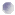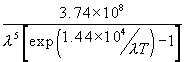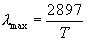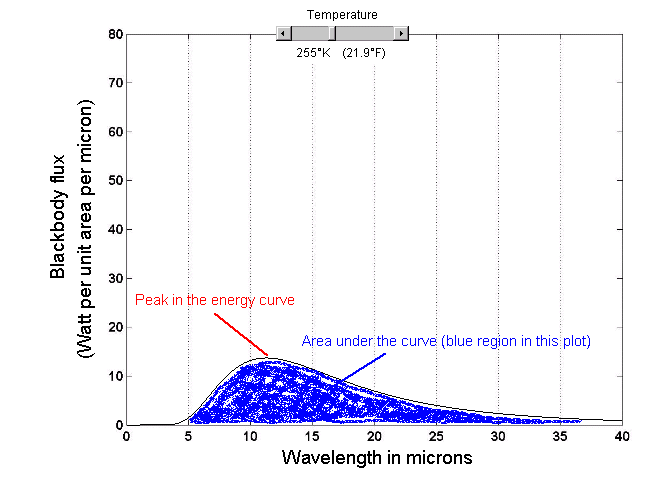### Meteorology: Understanding the Atmosphere            Ackerman and Knox

. Some objects emit and absorb radiation better than others. A blackbody is an object that absorbs all the electromagnetic energy that falls on the object, no matter what the wavelength of the radiation. A perfect blackbody does not exist, but it is a useful reference for determining how good a body is at emitting and absorbing radiation. While an object may visually appear black, it does not mean it is a blackbody. Using the applet below you can draw energy curves similar to that shown in Figure 2.10. The x-axis is wavelength in microns of the emitted radiation. The y-axis is the amount of radiant energy emitted at a give wavelength. The formulation assumes that the objects emitting the radiation are perfect blackbodies (more on this formulation).

As you adjust the temperature of the emitting object using the slider at the top of the figure, notice:How the area under the curve increases as the temperature is increased (The Stefan-Boltzmann Law),How the peak in the emitted energy changes wavelength (Wien's Law),For a perfect blackbody, energy is emitted at all wavelengths, the issue is how much energy is emitted.

Can you use this tool to explain why the distribution of emitted radiation with wavelength varies so differently between the Earth and the Sun?

### Planck's Law

The amount of blackbody radiative flux emitted by a blackbody for a given wavelength is given by Planck's Law:where T is the objects temperature (in degrees Kelvin) and l is the wavelength in microns, and the units are (W/m2) per micron. The wavelength of th epeak eission is### Area under the curve